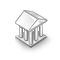Interesting script?
So post a link to it -
let others appraise it

You liked the script? Try it in the MetaTrader 5 terminal# dt_FFT - library for MetaTrader 5

Views:
4900
Rating:
Published:
2015.01.28 15:46
Updated:
2016.11.22 07:32

Real author:

klot

Library of fast Fourier transformation functions (FFT).

This library was first implemented in MQL4 and published in CodeBase on October 2, 2006.

The library has seven fast Fourier transformation functions:

1. FFT of a complex function (direct and inverse)
void fastfouriertransform(double& a[], int nn, bool inversefft);

The algorithm makes fast Fourier transformation of a complex function defined by nn counts on a real axis. Depending on the parameters passed, both direct or inverse transformation can be executed.

Input Parameters:

• nn - Number of values of the function. Must be a power of two!!! The algorithm doesn't check the correctness of the passed value.
• a - array [0 .. 2*nn-1] of Real. Values of the function. Elements a[2*I] (real part) and a[2*I+1] (imaginary part) correspond to the first value.
• InverseFFT - direction of transformation. True, if it's reverse; False, if it's direct.

Input parameters:

2. FFT of a real function (direct and inverse)
void realfastfouriertransform(double& a[], int tnn, bool inversefft);

The algorithm makes fast Fourier transformation of a real function defined by n counts on a real axis. Depending on  the parameters passed, both direct or inverse transformation can be executed.

Input Parameters:

• tnn - Number of values of the function. Must be a power of two!!! The algorithm doesn't check the correctness of the passed value.
• a - array [0 .. nn-1] of Real. Values of the function.
• InverseFFT - direction of transformation. True, if it's reverse; False, if it's direct.

Input parameters:

3. FFT of two real functions (direct only)
void tworealffts(double a1[], double a2[], double& a[], double& b[], int tn);

The algorithm makes fast Fourier transformation of two real functions each of which is defined by tn counts on a real axis. The algorithm saves your time but performs direct transformation only.

Input Parameters:

• tn - Number of values of the function. Must be a power of two. The algorithm doesn't check the correctness of the passed value.
• a1 - array [0 .. nn-1] of Real. Value of the first function.
• a2 - array [0 .. nn-1] of Real. Values of the second function.

Input parameters:

4. Fast discrete sine transformation
void fastsinetransform(double& a[], int tnn, bool inversefst);

The algorithm makes fast sine transformation of a real function defined by tnn counts on a real axis. Depending on the parameters passed, both direct or inverse transformation can be executed.

Input Parameters:

• nn - Number of values of the function. Must be a power of two. The algorithm doesn't check the correctness of the passed value.
• a - array [0 .. nn-1] of Real. Values of the function.
• InverseFST - direction of transformation. True, if it's reverse; False, if it's direct.

Input parameters:

5. Fast discrete cosine transformation
void fastcosinetransform(double& a[],int tnn, bool inversefct);

The algorithm makes fast cosine transformation of a real function defined by nn counts on a real axis. Depending on the parameters passed, both direct or inverse transformation can be executed.

Input Parameters:

• tnn - Number of values of the function minus one. Must be a power of two (for example 1024). The algorithm doesn't check the correctness of the passed value.
• a - array [0..nn] of Real. Function values (for example, 1025).
Peculiarity of the preparation of an array for function transfer:
int element_count2=ArrayResize(array,tnn1+1); //For cosine !!!
• InverseFCT - direction of transformation. True, if it's reverse; False, if it's direct.

Input parameters:

6. Fast simplification with FFT
void fastcosinetransform(double& a[],int tnn, bool inversefct);

Simplification. One of the functions is assumed as a signal. The second one is considered a response.

Input:

• Signal - a signal with which simplification is made. Array of real numbers, numbering of elements from 0 to SignalLen-1.
• SignalLen - signal length.
• Response - response function. It consists of two parts corresponding to positive and negative values of an argument.
Response values in points from -NegativeLen to 0 correspond to array elements with numbers from 0 to NegativeLen.
Response values in points from 1 to PositiveLen correspond to array elements with numbers from NegativeLen+ to NegativeLen+PositiveLen.
• NegativeLen - "Negative length" of a response.
• PositiveLen -"Positive length" of a response.
A response is equal to zero beyond [-NegativeLen, PositiveLen].

Output:

• Signal - values of function simplification in points from 0 to SignalLen-1.

7. Fast correlation with FFT
void fastcorellation(double& signal[], int signallen,  double& pattern[],  int patternlen);

Input:

• Signal - array signal with which correlation is made. Numbering of elements from 0 to SignalLen-1
• SignalLen - signal length.
• Pattern - array pattern correlation of a signal with which we are looking for. Numbering of elements from 0 to PatternLen-1
• PatternLen - pattern length

Output:

Translated from Russian by MetaQuotes Software Corp.
Original code: https://www.mql5.com/ru/code/7000FileUnlimited

Library for working with files using WinAPI without location limitations.Mutex - WinAPI

Synchronize ОС/EA/MT processes etc. Any self-made DLLs are not needed now.CHashArrayStringString

Example of implementation of lines hash array with a string key.Indicator Arrows II

Plots up/down buffer arrows in chart window.